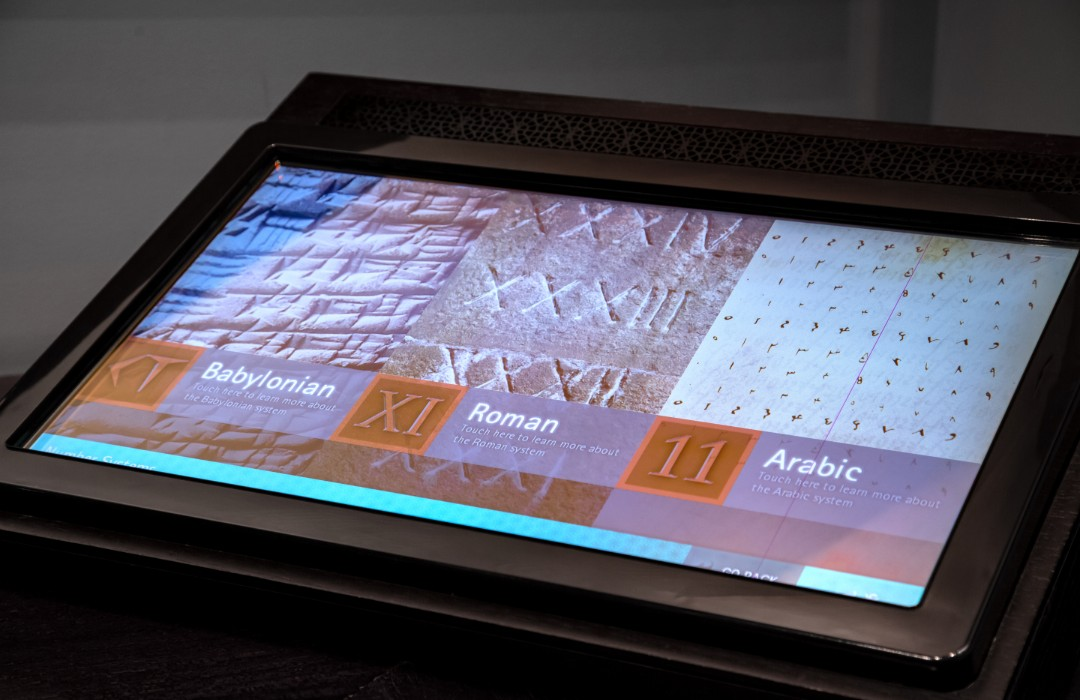# Explore the Museum / Mathematics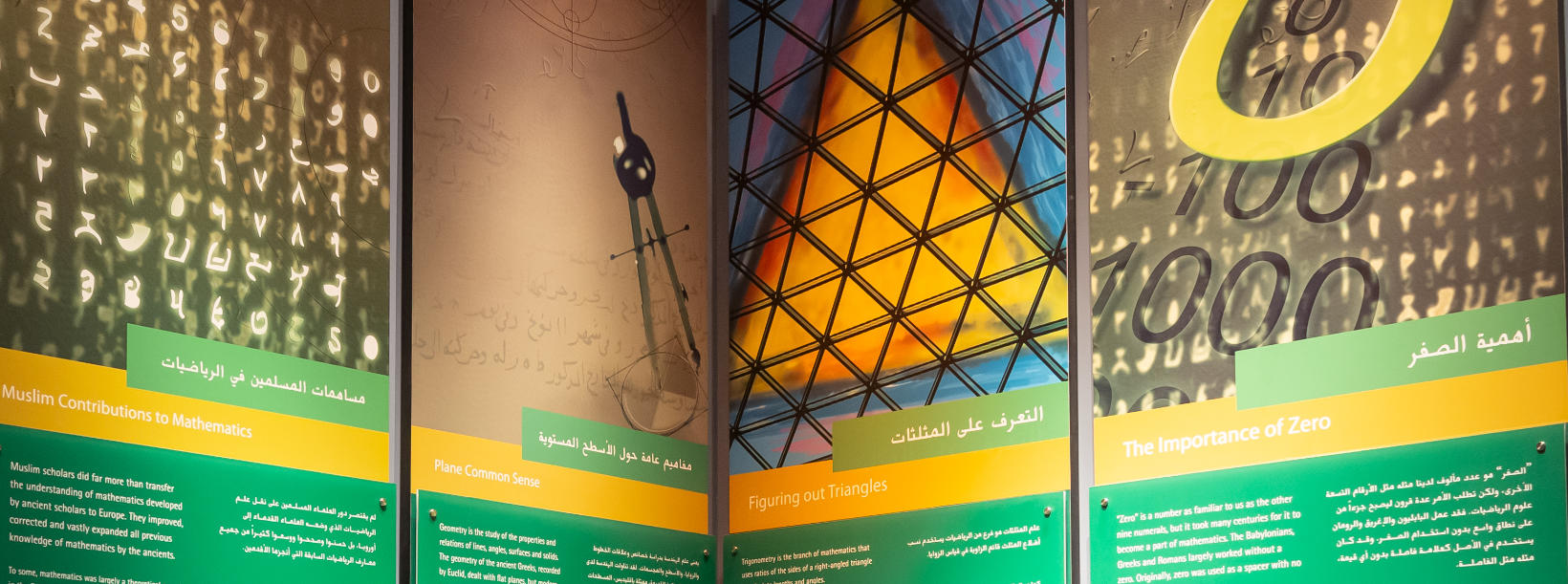The way in which Muslim mathematicians lead the development of arithmetic, geometry, trigonometry and algebra is explored in exciting interactive exhibits and games. In one game, the visitor compares the efficiency of doing arithmetic using Arabic, Babylonian and Roman numerals.

## Mathematics

This display allows visitors to explore aspects of Islamic contributions to mathematics, including the work of great Muslim mathematicians, developments in different fields of mathematics, definitions of mathematical terms, and the main divisions into which mathematics is divided.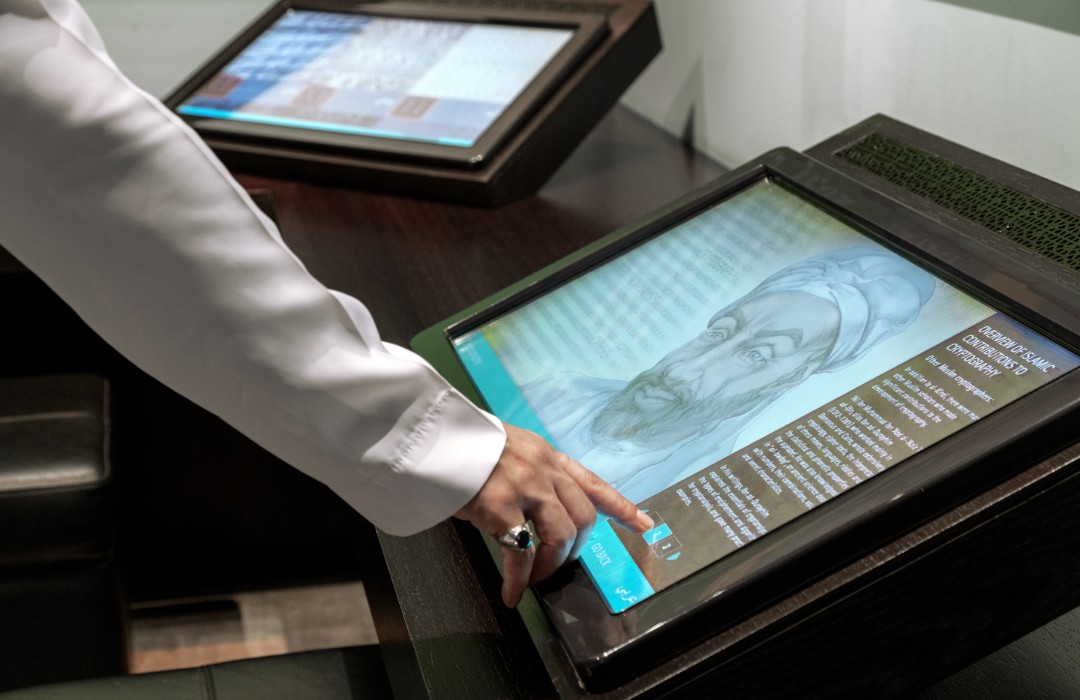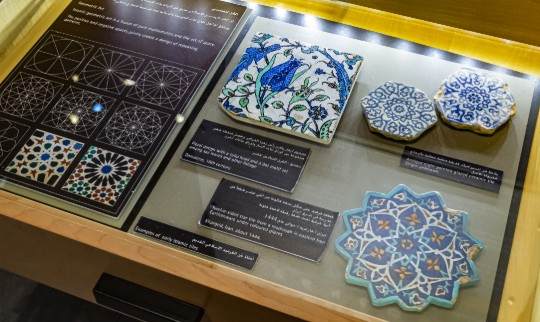## Geometric art

Examples of Islamic geometric art are shown in this display.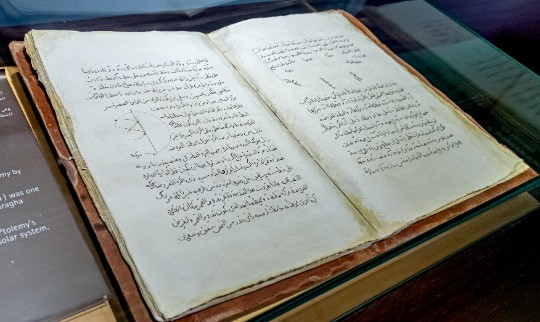## mathematics in astronomy

This display comprises a replica of a famous book on mathematics in astronomy by al-Tusi.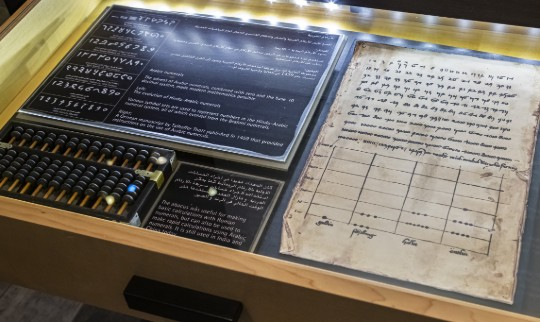## Development of Arabic numerals

The development of early Hindu numerals into ancient and modern Arabic numerals is shown in this display.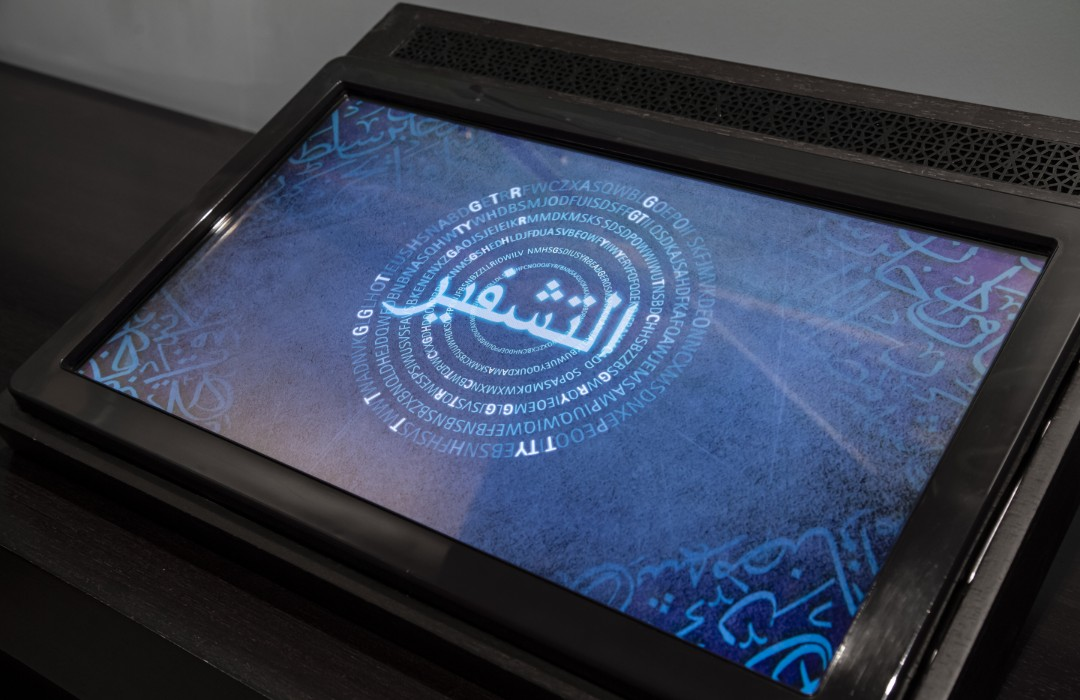## Cryptography

Al-Kindi and other early Islamic scholars had a very good grasp of mathematics, logic and language, and were the first to introduce complex encryption and decryption methods into cryptography, such as the Frequency Method.

Visitors are challenged to decode a simple encoded message.

## Different numeral systems

This interactive touch screen display helps you to understand how much easier it is to do arithmetic (addition, subtraction, division, multiplication) using modern Arabic numerals compared to ancient Babylonian or Roman numerals.